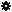﻿ Object Model: Vertex.GrowNeighborVertices

Vertex.GrowNeighborVertices

## Vertex.GrowNeighborVerticesv3.0

### Description

Returns a collection of Vertex objects for all vertices that are adjacent to this Vertex within the specified distance.

### C# Syntax

 ```VertexCollection Vertex.GrowNeighborVertices( Int32 in_lDistance ); ```

### Scripting Syntax

 ```oReturn = Vertex.GrowNeighborVertices( [Distance] ); ```

### Parameters

Parameter Type Description
Distance Integer Distance is an integer value representing the degree of neighborhood (eg: degree=2 for a vertex means its adjacent vertices plus the adjacent vertices of the adjacent vertices)

Default Value: 1

### Examples

#### VBScript Example

 ```set oCube = ActiveSceneRoot.AddGeometry( "Cube", "MeshSurface" ) set oGeometry = oCube.ActivePrimitive.Geometry for each Vertex in oGeometry.Vertices set oNeighbors = Vertex.GrowNeighborVertices str = "Vertices around Vertex(" & Vertex.index & ") are :" for each n in oNeighbors str = str & " " & n.index next logmessage str next ```